Excess Returns and Terminal Value

Stable growth firms tend to reinvest less than high growth firms and it is critical that we both capture the effects of lower growth on reinvestment and that we ensure that the firm reinvests enough to sustain its stable growth rate in the terminal phase. The actual adjustment will vary depending upon whether we are discounting dividends, free cash flows to equity or free cash flows to the firm.

In the dividend discount model, note that the expected growth rate in earnings per share can be written as a function of the retention ratio and the return on equity.

Expected Growth Rate = Retention ratio * Return on Equity

Algebraic manipulation can allow us to state the retention ratio as a function of the expected growth rate and return on equity:

Retention ratio =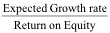If we assume, for instance, a stable growth rate of 5% (based upon the growth rate of the economy) for Procter & Gamble and a return on equity of 15% (based upon industry averages), we would be able to compute the retention ratio in stable growth:

Retention ratio =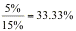Procter & Gamble will have to reinvest 33.33% of its earnings into the firm to generate its expected growth of 5%; it can pay out the remaining 66.67%.

In a free cash flow to equity model, where we are focusing on net income growth, the expected growth rate is a function of the equity reinvestment rate and the return on equity.

Expected Growth Rate = Equity Reinvestment rate * Return on Equity

The equity reinvestment rate can then be computed as follows:

Equity Reinvestment rate =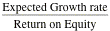If, for instance, we assume that Coca Cola will have a stable growth rate of 5.5% and that its return on equity in stable growth of 18%, we can estimate an equity reinvestment rate:

Equity Reinvestment rate =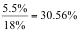Finally, looking at free cash flows to the firm, we estimated the expected growth in operating income as a function of the return on capital and the reinvestment rate:

Expected Growth rate = Reinvestment rate * Return on Capital

Again, algebraic manipulation yields the following measure of the reinvestment rate in stable growth.

Reinvestment Rate in stable growth =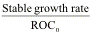where the ROCn is the return on capital that the firm can sustain in stable growth. This reinvestment rate can then be used to generate the free cash flow to the firm in the first year of stable growth.

Linking the reinvestment rate and retention ratio to the stable growth rate also makes the valuation less sensitive to assumptions about stable growth. While increasing the stable growth rate, holding all else constant, can dramatically increase value, changing the reinvestment rate as the growth rate changes will create an offsetting effect. The gains from increasing the growth rate will be partially or completely offset by the loss in cash flows because of the higher reinvestment rate. Whether value increases or decreases as the stable growth increases will entirely depend upon what you assume about excess returns. If the return on capital is higher than the cost of capital in the stable growth period, increasing the stable growth rate will increase value. If the return on capital is equal to the stable growth rate, increasing the stable growth rate will have no effect on value. This can be proved quite easily.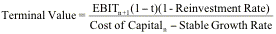Substituting in the stable growth rate as a function of the reinvestment rate, from above, you get: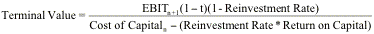Setting the return on capital equal to the cost of capital, you arrive at: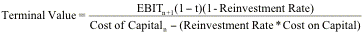Simplifying, the terminal value can be stated as: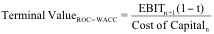You could establish the same proposition with equity income and cash flows and show that a return on equity equal to the cost of equity in stable growth nullifies the positive effect of growth.